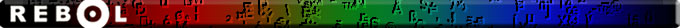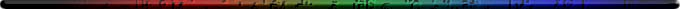Script Library: 1238 scripts

# Documentation for: regset.r# REGSET.r

Boleslav Brezovsky
31-5-2007

## Contents:

1. Introduction
2. Usage
2.1 Escaped characters
2.2 Predefined sequences
3. Examples
3.1 Usage with 'parse

## 1. Introduction

%regset.r is part of R2E2 - REBOL Regular Expressions Engine.

Purpose of 'regset is to convert small bit of regex to bitset! 'regset can handle regex' groups (everything in between [ and ].)

## 2. Usage

```>> regset "a-z"
== make bitset! #{
000000000000000000000000FEFFFF0700000000000000000000000000000000
}
```

You can write a letter ("a"), a range ("a-z"), or you can exclude a set using "~" or "^".

### 2.1 Escaped characters

Escaped character must be escaped using "\" (back-slash). Following chars need to be escaped:

```. ^ \$ * + ? { [ ] \ | ( )
```

Other escaped characters (typically whitespaces):

```\t - TAB
\r - CR
\n - LF
\a - bell
\e - escape
\f - form feed
\v - vertical tab
```

### 2.2 Predefined sequences

'regset supports some predefined sqeunces:

• \d

Matches any decimal digit; this is equivalent to the class [0-9].

• \D

Matches any non-digit character; this is equivalent to the class [^0-9].

• \s

Matches any whitespace character; this is equivalent to the class [ \t\n\r\f\v].

• \S

Matches any non-whitespace character; this is equivalent to the class [^ \t\n\r\f\v].

• \w

Matches any alphanumeric character; this is equivalent to the class [a-zA-Z0-9_].

• \W

Matches any non-alphanumeric character; this is equivalent to the class [^a-zA-Z0-9_].

## 3. Examples

### 3.1 Usage with 'parse

• parse a word (alphanum characters)
```>> parse "chleba" compose [some (regset "\w")]
== true
```
• parse a phone number
```>> parse "#340-5464" reduce ["#" 3 regset "\d" "-" 4 regset "\d"]
== true
```MakeDoc2 by REBOL- 31-May-2007